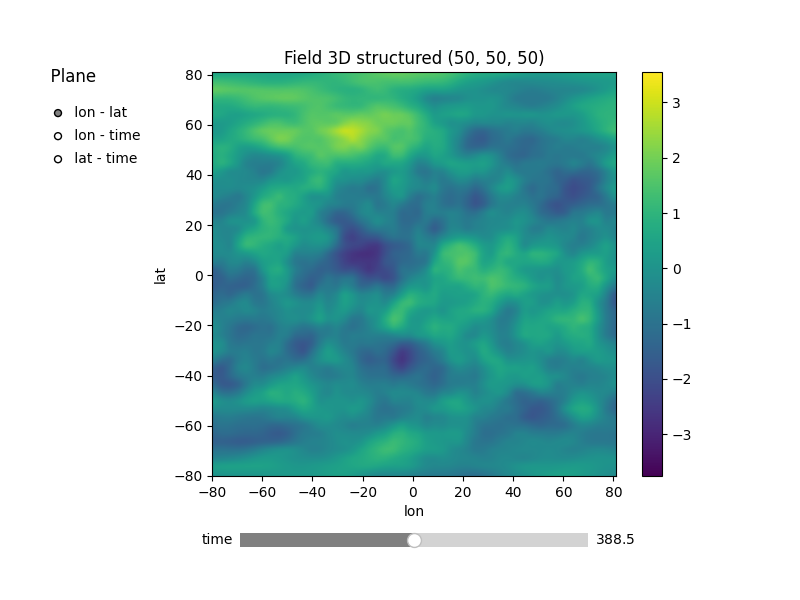# Working with spatio-temporal lat-lon fields

In this example, we demonstrate how to generate a spatio-temporal random field on geographical coordinates.

First we setup a model, with `latlon=True` and `temporal=True`, to get the associated spatio-temporal Yadrenko model.

In addition, we will use a kilometer scale provided by `KM_SCALE` as `geo_scale` to have a meaningful length scale in km. By default the length scale would be given in radians (`RADIAN_SCALE`). A third option is a length scale in degrees (`DEGREE_SCALE`).

To generate the field, we simply pass `(lat, lon, time)` as the position tuple to the `SRF` class.

We will set a spatial length-scale of 1000 and a time length-scale of 100 days.```import numpy as np

import gstools as gs

model = gs.Matern(
latlon=True,
temporal=True,
var=1,
len_scale=[1000, 100],
geo_scale=gs.KM_SCALE,
)

lat = lon = np.linspace(-80, 81, 50)
time = np.linspace(0, 777, 50)
srf = gs.SRF(model, seed=1234)
field = srf.structured((lat, lon, time))
srf.plot()
```

Total running time of the script: ( 0 minutes 8.315 seconds)

Gallery generated by Sphinx-Gallery